# ISEE Upper Level Quantitative : How to find the length of a side

## Example Questions

### Example Question #1 : How To Find The Length Of A Side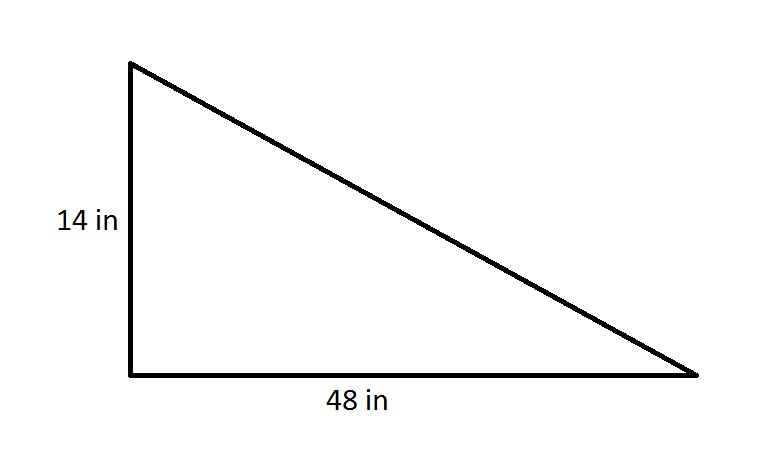A regular decagon has the same perimeter as the above right triangle. Give the length of one side.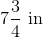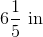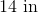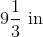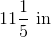Explanation:

By the Pythagorean Theorem, the hypotenuse of the right triangle is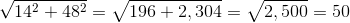inches, making its perimeter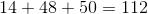inches.

A regular decagon has ten sides of equal length, so each side measures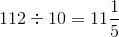inches.

### Example Question #1 : How To Find The Length Of A Side

A regular octagon has perimeter one meter. Which is the greater quantity?

(A) The length of one side

(B) 125 millimeters

(B) is greater

(A) and (B) are equal

(A) is greater

It is impossible to determine which is greater from the information given

(A) and (B) are equal

Explanation:

A regular octagon has eight sides of equal length. The perimeter of this octagon is one meter, which is equal to 1,000 millimeters; each side, therefore, has length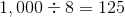millimeters

making the quantities equal.

### Example Question #2 : How To Find The Length Of A Side

A regular pentagon has sidelength 72; the perimeter of a regular hexagon is 80% of that of the pentagon. Which is the greater quantity?

(A) The length of one side of the hexagon

(B) 50

(A) is greater

It is impossible to determine which is greater from the information given

(B) is greater

(A) and (B) are equal

(B) is greater

Explanation:

A regular pentagon has five sides of equal length; since one side is 72 units long, its perimeter is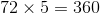.

80% of this is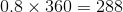,

so this is the length of the hexagon, and, since all six sides are of equal length, one side measures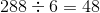(B) is greater.

### Example Question #4 : How To Find The Length Of A Side

A regular octagon has twice the perimeter of a regular pentagon. What is the ratio of the sidelength of the octagon to that of the pentagon?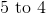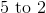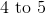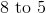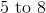Explanation:

The solution is independent of the actual lengths, so we assume the pentagon has sidelength 1. Its perimeter is therefore 5. Subsequently, the octagon's perimeter is twice this, or 10, and its sidelength is one-eighth of this, or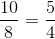.

The ratio of the sidelength of the octagon to that of the pentagon is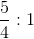or 5 to 4.

### Example Question #2 : How To Find The Length Of A Side

A regular octagon has perimeter one mile.Which is the greater quantity?

(a) The length of one side

(b) 880 feet

It is impossible to determine which is greater from the information given

(a) and (b) are equal

(a) is the greater quantity

(b) is the greater quantity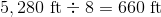. This makes the length of a side less than 880 feet.